This is the supplementary blog article describing how to manipulate the alert words at various scenario or conditions for TradingView indicators and strategies.

Keywords to close SHORT trade: closesell,closeshort,close sell,sell close,close short,short close,exit sell,sell exit,exit short,short exit

If you want to close all trades, set keywords list to 'Keywords to close both LONG & SHORT trades'.

Below suggestions will first go through TradingView indicator alerts and then strategy's.

There are two methods to write pinescript alert codes:

and

if you're using 'alert' function, i share one sample code snippet as below.  It will simply raise an BUY alert if a bullish candle bar gets closed or SELL if bearish.  You could put this sample alert indicator on an one minute chart for fast trigger.

```//@version=5

indicator(title = "Indicator alert test", overlay = true)

sigSell = close < open

if sigSell

plot(close, color = na)```

This example will trigger an alert after a bar close.  If you want that alert to be raised at realtime mode, just simply write alert("Bullish candle, BUY")  Pinescript 'alert' default frequency is to trigger once a bar.

If you prefer using 'alertcondition', it will look like as below codes - and you will be able to choose what conditions to be alerted at setting:

```//@version=5

indicator(title = "Indicator alertcondition test", overlay = true)

conSell = close < open

alertcondition(conSell, title = "Sell Signal", message = "Bearish candle, SELL")

plot(close, color = na)```

But please be aware the default alert frequency of 'alertcondition' is only raised ONCE.  It's essential to change this setting to 'Once Per Bar' or 'Once Per Bar Close' if you want continuous alerts.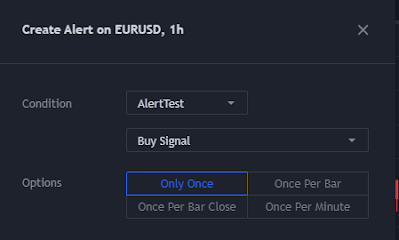Below are my pinescript code snippet showing how to add trade entry/exit actions by using order 'comment'.

```//@version=5
strategy(title = "Strategy Alert Test - Market Order", overlay = true)

lot = input.int(title = "Trade lot", defval = 100, minval = 1)
startDate = input.time(title = "Start date", defval = timestamp('2022-01-01'))

bool inDateRange = time >= startDate

rrr = 2.0
atrMulti = 3.0
atrVal = ta.atr(50)

maShort = ta.sma(close, 50)
maLong = ta.sma(close, 100)
sigSell = ta.crossunder(maShort, maLong)
plot(maShort, color = color.blue)
plot(maLong, color = color.red)

strategy.entry(id = "buy", direction = strategy.long, qty = lot, comment = "buy")
slPrice = math.round_to_mintick(close + syminfo.mintick * 1 - atrVal * atrMulti)
tpPrice = math.round_to_mintick(close + syminfo.mintick * 1 + atrVal * atrMulti * rrr)
strategy.exit(id = "buy exit", from_entry = "buy", stop = slPrice, limit = tpPrice, comment = "close buy")
strategy.entry(id = "sell", direction = strategy.short, qty = lot, comment = "sell")
slPrice = math.round_to_mintick(close + atrVal * atrMulti)
tpPrice = math.round_to_mintick(close - atrVal * atrMulti * rrr)
strategy.exit(id = "sell exit", from_entry = "sell", stop = slPrice, limit = tpPrice, comment = "close sell")```

The words of order comments are in fact the exact texts shown on TradingView chart for each trade action.  Or you could use 'alert_message' feature at strategy.entry or strategy.exit as well.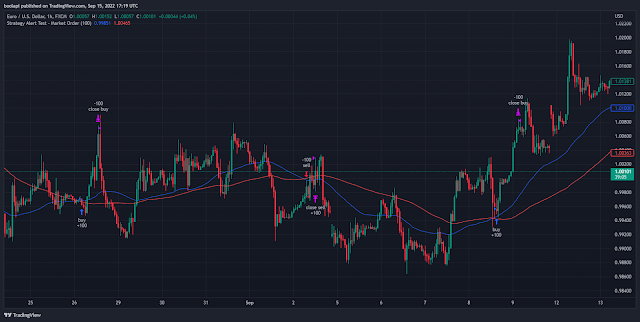This sample strategy takes the up or down cross of two simple moving average lines as buy and sell signals.  Stoploss price is the 3 times of atr values (atr period = 50).  Take profit price is based on the 'risk reward ratio' = 2.0.

Then you will need to input

{{strategy.order.comment}}

If you're using 'alert_message' feature other than 'comment', here it should be {{strategy.order.alert_message}} at this setting.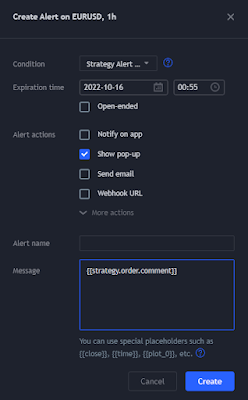Using 'comment' word is still okay for this order fulfill scenario.  Anyway I make below sample codes for stop order scenario and put the message words at 'alert_message' part.

The stop price is 1.0 times of atr value - and stoploss and take profit rules follow the previous example.

```//@version=5
strategy(title = "Strategy Alert Test - Stop Order", overlay = true)

lot = input.int(title = "Trade lot", defval = 100, minval = 1)
startDate = input.time(title = "Start date", defval = timestamp('2022-01-01'))

bool inDateRange = time >= startDate

rrr = 2.0
atrMultiOpen = 1.0
atrMultiSp = 3.0
atrVal = ta.atr(50)

maShort = ta.sma(close, 50)
maLong = ta.sma(close, 100)
sigSell = ta.crossunder(maShort, maLong)
plot(maShort, color = color.blue)
plot(maLong, color = color.red)

openPr = math.round_to_mintick(close + syminfo.mintick + atrVal * atrMultiOpen)
slPrice = math.round_to_mintick(openPr - atrVal * atrMultiSp)
tpPrice = math.round_to_mintick(openPr + atrVal * atrMultiSp * rrr)
if sigSell and inDateRange
openPr = math.round_to_mintick(close - syminfo.mintick + atrVal * atrMultiOpen)
slPrice = math.round_to_mintick(openPr + atrVal * atrMultiSp)
tpPrice = math.round_to_mintick(openPr - atrVal * atrMultiSp * rrr)
strategy.entry("sell", direction = strategy.short, stop = openPr, alert_message = "Sell")
strategy.exit(id = "sell exit", from_entry = "sell", stop = slPrice, limit = tpPrice, alert_message = "close sell")
```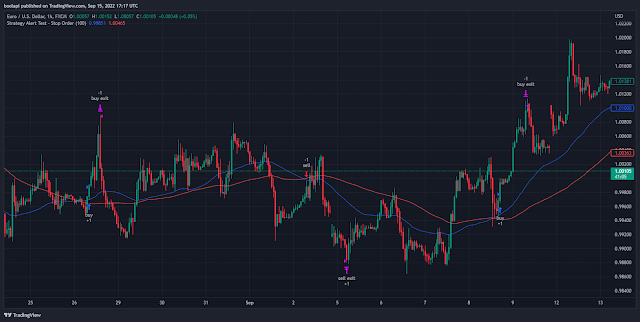As 'alert_message' feature is now used at codes, the alert setting should have {{strategy.order.alert_message}} so the exact words will show as alert texts.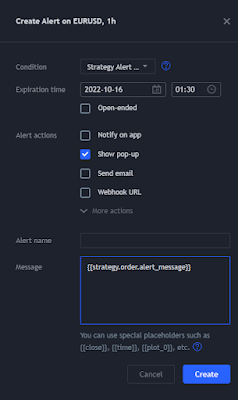Partial close

If you want to have partial close actions, just add xx% at strategy exit alert text, e.g. 'close long 50%'.

Below is the sample pinescript codes you could use as reference.  At MT4/MT5 copier ea, you don't need to do any setting.

```//@version=5
strategy(title = "Strategy Partial Close", overlay = true, calc_on_order_fills = false, initial_capital = 100000, currency = "USD")

lot = input.int(title = "Trade lot", defval = 100, minval = 1)
isSell = input.bool(title = "Sell?", defval = true)
startDate = input.time(title = "Start date", defval = timestamp('2022-11-15'))

bool inDateRange = time >= startDate

buySig = close < open and close > open
buyPCSig = close > open and close > open
buyACSig = close > open and close > open and close > open

sellSig = close > open and close < open
sellPCSig = close < open and close < open
sellACSig = close < open and close < open and close < open

var bool _sellPartial = false

strategy.entry(id = "buy", direction = strategy.long, qty = lot, comment = "buy")

if isSell and sellSig and inDateRange and strategy.position_size >= 0
strategy.entry(id = "sell", direction = strategy.short, qty = lot, comment = "sell")
_sellPartial := true
if isSell and sellPCSig and inDateRange and strategy.position_size < 0 and _sellPartial
strategy.close(id = "sell", qty_percent = 50, comment = "close sell 50%")
_sellPartial := false
if isSell and sellACSig and inDateRange and strategy.position_size < 0
strategy.close(id = "sell", comment = "close sell")```

#
Share it with friends: